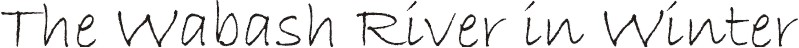VBA Macros for Solving Problems in Water Chemistry

This web page contains links to an Adobe Acrobat formatted text file that contains instructions for writing Visual Basic for Application (VBA) macros within Microsoft Excel (i.e., the application), and several Excel spreadsheets that contain sample VBA programs.  Upon opening each spreadsheet you will need to 'enable macros' before they can be executed (i.e., run).  All the macros are linked to ActiveX control buttons on the spreadsheets, making them easy to run.  The author retains all copyrights.

This Acrobat file contains the instructions for writing VBA macros in Excel and further explanations on each of the sample spreadsheets below. (15 pages)

Instructions

An Excel spreadsheet that contains an example VBA macro that shows how to: (i) dimension variables and arrays, (ii) write 'For .  .  . Next' loops, (iii) read and write to the spreadsheet, (iv) format common mathematical expressions, and (v) do other common programming operations.

A simple spreadsheet than contains some user-defined functions (volume of a cylinder, roots to the quadratic equation, and the sin of an angle measured in degrees).

A spreadsheet that calculates buffer composition and draws the pC-pH diagram of a simple acid.

A spreadsheet that solves a system of three equations and three unknowns by Newton-Raphson iterations.  The 'recipe' for the problem is the same as 'Case 3' on page 60-63 in the text "Principles and Applications of Aquatic Chemistry" by Morel and Hering.

A spreadsheet that solves a system of 2 simultaneous ordinary differential equations (ODE's) with Euler's method.  The example case is the transport of tetrachloroethylene in a stratified lake - Lake Greifensee, Switzerland - presented on pages 551-574 in "Environmental Organic Chemistry" by Schwarzenbach, Gschwend & Imboden.  The 2 ODE's are eqs 15-30a and 15-30b (p. 569) in the text.

Stratified Lake.xls

The central difference solution to the 1-D diffusion equation with an impulse input at the center of the media.  The numerical solution is calculated with a VBA macro and is compared to the analytical solution calculated directly on the spreadsheet.  A second macro calculates the mass under the concentration profile with Simpson's Method.

1D Diffusion.xlsOther files: